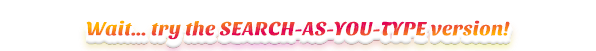# Group Data

In pandas group by involves one or more of the following steps:

• splitting the data into groups based on some criteria
• applying a function to each group independently (i.e aggregation, transformation, filtration)
• combining the results into a data structure (based on apply operation type)

## Group by one column, one column aggregated, one aggregation

# returns series because we aggregate one column
df.groupby('col_grp_1')['col_agg_1']\
.min()


## Group by two columns, one column aggregated, one aggregation

# returns series because we aggregate one column
df.groupby(['col_grp_1', 'col_grp_2'])['col_agg_1']\
.min()


## Group by two columns, multiple columns aggregated, one aggregation

# returns data frame because we aggregate multiple columns, one aggregation
df.groupby(['col_grp_1', 'col_grp_2'])['col_agg_1', 'col_agg_2']\
.min()


## Group by one column, one column aggregated, multiple aggregations

# returns data frame because of multiple aggregations
df.groupby('col_grp_1')['col_agg_1'].agg(['min', 'max', 'mean'])


## Group by one column, multiple columns aggregated, multiple aggregations

# returns data frame with hierarchical index in columns
df1 = df.groupby('col_grp_1')['col_agg_1', 'col_agg_2']\
.agg(['min', 'max', 'mean', 'count'])
# flatten the hierarchical index in columns
df1.columns = ['_'.join(col).strip() for col in df1.columns.values]


## Group by named aggregation: group by one column, multiple columns aggregated, multiple aggregations

# from pandas version >= 0.25.0
df.groupby('col_grp_1')\
.agg(new_col1_name=('col_agg_1', 'mean'),
new_col2_name=('col_agg_2', 'count'))


## Group by one column, one column aggregated, one aggregation

# giving DataFrame df
>>> df
kind      breed  height  weight
0  cat    siamese     9.1     7.9
1  dog  chihuahua     6.0     7.5
2  cat    persian     9.5     9.9
3  dog   labrador    34.0   198.0
4  dog  chihuahua     3.5     5.5

# returns series because we aggregate one column
df.groupby('kind')['weight'].min()

kind
cat    7.9
dog    5.5
Name: weight, dtype: float64


## Group by two columns, one column aggregated, one aggregation

# giving DataFrame df
>>> df
kind      breed  height  weight
0  cat    siamese     9.1     7.9
1  dog  chihuahua     6.0     7.5
2  cat    persian     9.5     9.9
3  dog   labrador    34.0   198.0
4  dog  chihuahua     3.5     5.5

# returns series because we aggregate one column
df.groupby(['kind', 'breed'])['weight'].min()

kind  breed
cat   persian        9.9
siamese        7.9
dog   chihuahua      5.5
labrador     198.0
Name: weight, dtype: float64


## Group by two columns, multiple columns aggregated, one aggregation

# giving DataFrame df
>>> df
kind      breed  height  weight
0  cat    siamese     9.1     7.9
1  dog  chihuahua     6.0     7.5
2  cat    persian     9.5     9.9
3  dog   labrador    34.0   198.0
4  dog  chihuahua     3.5     5.5

# returns data frame because we aggregate multiple columns, one aggregation
df.groupby(['kind', 'breed'])['height', 'weight'].min()

height  weight
kind breed
cat  persian       9.5     9.9
siamese       9.1     7.9
dog  chihuahua     3.5     5.5
labrador     34.0   198.0


## Group by one column, one column aggregated, multiple aggregations

# giving DataFrame df
>>> df
kind      breed  height  weight
0  cat    siamese     9.1     7.9
1  dog  chihuahua     6.0     7.5
2  cat    persian     9.5     9.9
3  dog   labrador    34.0   198.0
4  dog  chihuahua     3.5     5.5

df.groupby('kind')['weight'].agg(['min', 'max', 'mean'])

# returns data frame because of multiple aggregations
min    max       mean
kind
cat   7.9    9.9   8.900000
dog   5.5  198.0  70.333333


## Group by one column, multiple columns aggregated, multiple aggregations

# giving DataFrame df
>>> df
kind      breed  height  weight
0  cat    siamese     9.1     7.9
1  dog  chihuahua     6.0     7.5
2  cat    persian     9.5     9.9
3  dog   labrador    34.0   198.0
4  dog  chihuahua     3.5     5.5

# returns data frame with hierarchical index in columns
df1 = df.groupby('kind')['weight', 'height']\
.agg(['min', 'max', 'count'])

# flatten the hierarchical index in columns
df1.columns = ['_'.join(col).strip() for col in df1.columns.values]
print(df1)

weight_min  weight_max  weight_count  height_min  height_max  height_count
kind
cat          7.9         9.9             2         9.1         9.5             2
dog          5.5       198.0             3         3.5        34.0             3


## Filter (within a GroupBy), filters the rows on a property of the group they belong to

# will return a subset DataFrame of rows belonging to the groups satisfying condition
df1 = df.groupby('col_grp').filter(lambda x : x['col'].mean() > 20)


## Transform (within a GroupBy), calculates a new value for each row based on a property of the group

df['grp_mean'] = df.groupby('col_grp')['col'].transform(lambda x : x.mean())


## Center the data by subtracting the group-wise mean

# will return a new DataFrame with same size as the original
df_new = df.groupby('col_grp').transform(lambda x: x - x.mean())


## Apply (within a GroupBy), applies an arbitrary function to the group results

def func_normalize(x):
# x is a DataFrame of group values
x['col1'] = x['col1'] / x['col2'].sum()

return x

# normalizes the first column by the sum of the second:
df = df.groupby('col_grp').apply(func_normalize)


## Get top n rows for each group of columns in a sorted dataframe

# sort DataFrame first by some columns
df = df.sort_values(['col_x','col_y'])
df_top_5 = df.groupby('col_x').head(5)


## Get bottom n rows for each group of columns in a sorted dataframe

# sort DataFrame first by some columns
df = df.sort_values(['col_x','col_y'])
df_bottom_5 = df.groupby('col_x').tail(5)